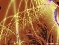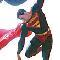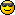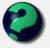Math Is Fun Forum

Discussion about math, puzzles, games and fun.   Useful symbols: ÷ × ½ √ ∞ ≠ ≤ ≥ ≈ ⇒ ± ∈ Δ θ ∴ ∑ ∫  π  -¹ ² ³ °

You are not logged in.

#1 2005-05-06 23:30:48

Milos
MemberRegistered: 2005-05-06
Posts: 44

Prove this

Prove that if the sides of a triangle are prime numbers its surface can not be whole number.

Try to work this out - it is interesting and not too difficult.
I will give you the possible solution in few days.

Offline

#2 2005-05-06 23:57:09

MathsIsFunRegistered: 2005-01-21
Posts: 7,710

Re: Prove this

Thank you Milos, something for us to think about ...

I might be able to get this STARTED, because there is a formula for working out the area of a triangle from its three side, it is called "Heron's Forumula", after a Greek mathematican called Heron who lived about 2000 years ago.

The formula is:

Area of Triangle = sqrt( s(s-a)(s-b)(s-c) )

Where a, b and c are the lengths of the three sides, and s (a+b+c)/2 (ie s=half the perimeter of the triangle)

But I haven't figured what to do next ....

"The physicists defer only to mathematicians, and the mathematicians defer only to God ..."  - Leon M. Lederman

Offline

#3 2005-05-07 03:34:18

Milos
MemberRegistered: 2005-05-06
Posts: 44

Re: Prove this

Yes, the idea is good, you are on the right way. Now we need proofs.:)

Offline

#4 2005-05-07 20:40:24

Roraborealis
MemberRegistered: 2005-03-17
Posts: 1,594

Re: Prove this

What if all the sides are 2cm (presuming it's an equilateral) then we do 2x2 divided by two and the answer is two? Two is a whole number.

School is practice for the future. Practice makes perfect. But - nobody's perfect, so why practice?

Offline

#5 2005-05-08 06:03:13

Milos
MemberRegistered: 2005-05-06
Posts: 44

Re: Prove this

If the triangle is equilateral then his angles are
180:3=60, and formula for surface you mentioned is for right-angled triangle.
If a=b=c=2 than P=(2xh)/2 . You can calculate h. cos30=h/a ; h=sqr3. So P=sqr3 - as you see this is not a whole number. This also could be the first proof.
But you should try to do it using Heron's formula which administrator mentioned.

Offline

#6 2005-05-10 09:59:17

Mr T
MemberRegistered: 2005-03-30
Posts: 1,012

Re: Prove this

ye but we're stupid

I come back stronger than a powered-up Pac-ManI bought a large popcorn @ the cinema the other day, it was pretty big...some might even say it was "largeFatboy Slim is a LegendOffline

#7 2005-05-30 23:09:02

Mr T
MemberRegistered: 2005-03-30
Posts: 1,012

Re: Prove this

why has no-one replied

I come back stronger than a powered-up Pac-ManI bought a large popcorn @ the cinema the other day, it was pretty big...some might even say it was "largeFatboy Slim is a LegendOffline

#8 2005-06-24 04:21:40

mathsyperson
ModeratorRegistered: 2005-06-22
Posts: 4,900

Re: Prove this

Substitute in s = (a+b+c)/2:

A = sqrt( ((a+b+c)/2)((a+b+c)/2-a)((a+b+c)/2-b)((a+b+c)/2-c) )
Yuck.
Simplifying: A= sqrt( (a+b+c)(-a+b+c)(a-b+c)(a+b-c)/16)
Take out the denominator: A=sqrt ((a+b+c)(-a+b+c)(a-b+c)(a+b-c)/4

I think I've taken the wrong route, but anyway...

Multiply out the brackets: A= sqrt((-a^4+2a^2*b^2+2a^2*c^2+2b^2*c^2-b^4-c^4))/4
Quite a few things cancelled out there, or it would be even more horrible!

To prove that A isn't a whole number we need to prove that the horrible thing inside the brackets isn't 4 times a square number.
Anyone?

Why did the vector cross the road?
It wanted to be normal.

Offline

#9 2005-06-25 04:57:52

NIH
MemberRegistered: 2005-06-14
Posts: 33

Re: Prove this

You're almost there, mathsyperson!

It's better to leave the expression as A^2 = (a+b+c)(-a+b+c)(a-b+c)(a+b-c)/16.  If a, b, c are all odd then the numerator is the product of four odd numbers, and hence is itself odd.  So A^2 is not an integer, and neither is A.  The same argument applies if two of a, b, c are equal to 2 and the remaining side length is odd.

Milos has already shown that if a = b = c = 2, then A = sqrt(3).  If a = 2 and b, c are odd, then, to form a triangle, we must have b = c, with b > 1.  (Try drawing a triangle with sides 2, 9, 11!)  So we have an isosceles triangle with base 2 and height sqrt(b^2 - 1).  b^2 - 1 is not a perfect square for b > 1, so the height is irrational, and hence also the area.

So we've proved a stronger result, a triangle with all sides lengths equal to either 2 or an odd number does not have integral area.

2 + 2 = 5, for large values of 2.

Offline